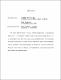## On the Difficulty of Breaking Substitution CiphersWe analyze different methods of attacking substitution ciphers using $m$-gram frequency analysis. For $m=1$ this amounts to studying symbol counts in random strings, and for $m\geq 2$ we use the Markov Chain Monte Carlo method introduced by Diaconis \cite{mcmcr}. Our study includes both numerical simulations of the English language and theoretical analysis of random alphabets, which are probabilistic constructions for studying the distribution of $m$-grams in random strings. We present several results in the direction of explaining why the $2$-gram method performs the best in breaking the substitution ciphers.